Create an Account

Home / Questions / Given is the following circuit Find analytically the impulse response h t of the system where

Given is the following circuit Find analytically the impulse response h t of the system where

Given is the following circuit. Find analytically the impulse response h(t) of the system where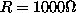and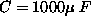.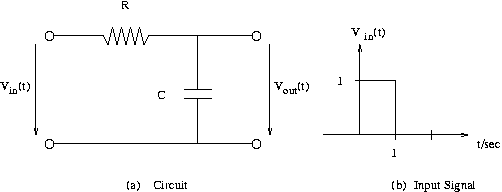Vin(t)=1 for 0 <= t <= 1sec. and 0 otherwise.

We obtain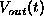by convolving the impulse response and the given input signal.

1.1 What is the analytical expression for the impuse response h(t) for t>0?
Use "^" to show a number raised to a power. (x squared would be x^2)

1.2 What is the analytical expression for the Vout for time, t<0?

1.3 What is the analytical expression for the Vout from time, t=0 to t=1sec?

1.4 What is the analytical expression for the Vout for time t>1sec?

1.5 Which of the following is the correct graph of Vout vs. time?
(a)
(b)
(c)
(d)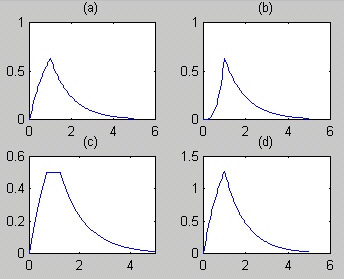View less »

Jul 31 2020 View more View LessSubscribe To Get Solution